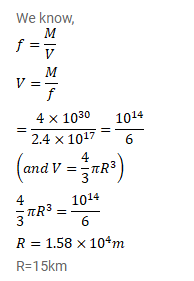# A neutron star has a density equal to that of a nuclear matter.

Question:

A neutron star has a density equal to that of a nuclear matter. Assuming the star to be spherical, find the radius of a neutron star whose mass $4.0 \times 10^{30} \mathrm{~kg}$ (twice the mass of the sun).

Solution: